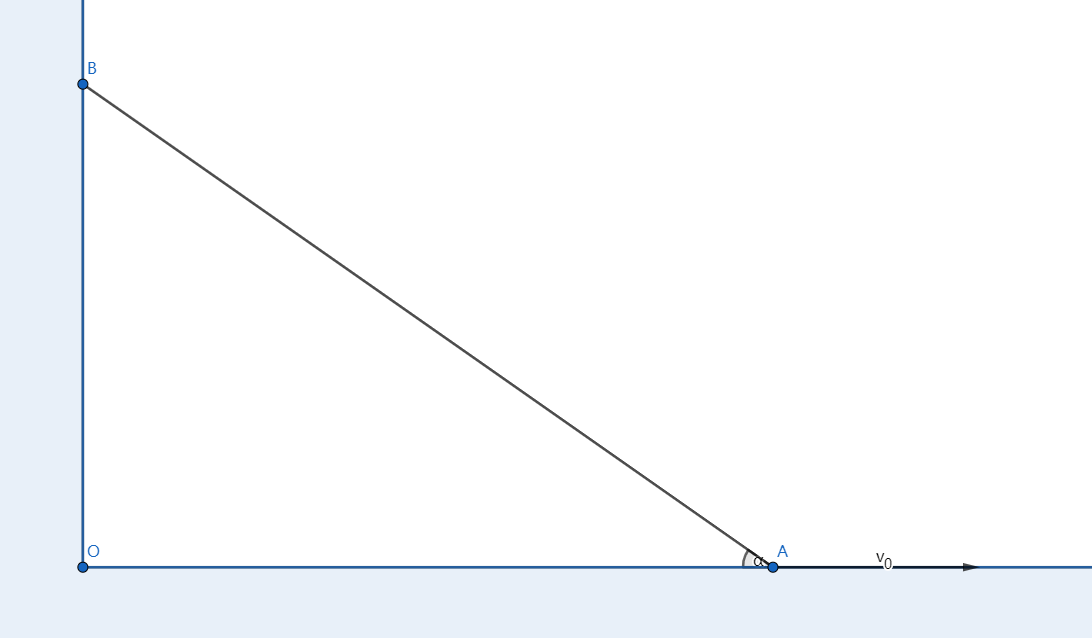# Reach for the Summit - P-S1-A2As shown above, a rod with length $l$ is leaning against the vertical wall. The lowest point of the rod, $A$ is moving right at velocity $v_0$, at this time, the angle of the rod and the ground is $\alpha$.

Then there exists a point on the rod which has the minimum magnitude of velocity. Find the minimum velocity.

Take $\alpha=30 \degree$, and the minimul velocity is $\lambda v_0$. Submit $\lfloor 10000 \lambda \rfloor$.

Reach for the Summit problem set - Physics

×